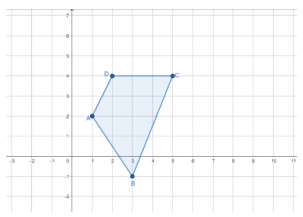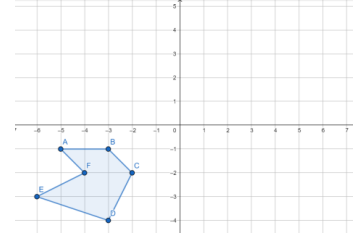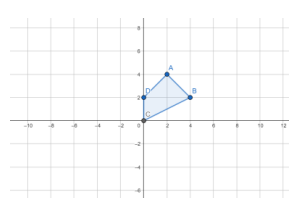# MA.912.GR.2.5Export Print
Given a geometric figure and a sequence of transformations, draw the transformed figure on a coordinate plane.

### Clarifications

Clarification 1: Transformations include translations, dilations, rotations and reflections described using words or using coordinates.

Clarification 2: Instruction includes two or more transformations.

General Information
Subject Area: Mathematics (B.E.S.T.)
Strand: Geometric Reasoning
Status: State Board Approved

## Benchmark Instructional Guide

### Terms from the K-12 Glossary

• Coordinate Plane
• Dilation
• Origin
• Reflection
• Rigid Transformation
• Rotation
• Scale Factor
• Translation

### Vertical Alignment

Previous Benchmarks

Next Benchmarks

### Purpose and Instructional Strategies

In grade 8, students learned about the effects of translations, rotations, reflections, and dilations on geometric figures. In Algebra 1, students extended this knowledge of transformations to transforming functions using tables, graphs and equations. In Geometry, students are given the preimage and a sequence of two or more transformations (a composition) and draw the image on a coordinate plane. In later courses, types of transformations will be expanded to include stretches that can transform functions and conic sections and conversions between rectangular and polar coordinates.
• The purpose of this benchmark is that students produce an image on the coordinate plane as the result of combining transformations (translations, dilations, rotations and reflections).
• Instruction includes transformations described using words and using coordinates.
• Instruction includes understanding that a single transformation can be done as a sequence of transformation. Likewise, some sequences can be described as a single transformation.
• For example, if the transformation sequence is to translate 1 unit to the left, then rotate 90-degrees clockwise about the origin, then translate 1 unit to the right; the result is the same as rotating the figure 90-degrees clockwise about the point (1,0). This can be described in coordinates as ($x$, $y$) → ($x$ − 1, $y$), then ($x$ − 1, $y$) → ($y$, −($x$ − 1)), then ($y$, −($x$ − 1)) → ($y$ + 1, −($x$ − 1)), and the result of rotating the figure 90-degrees clockwise about the point (1,0) can be described in coordinates as ($x$, $y$) → ($y$ + 1, −$x$ + 1).
• Instruction includes discussing the resulting images using the same preimage and a set of transformations in different orders and understanding that transformations may or not be commutative based on the sequence and type of transformations.
• For example, if the transformation is the result of a vertical and a horizontal translation, the sequence is commutative.
• For example, if the transformation is the result of a 180° rotation and a reflection over the $x$-axis, the sequence is commutative.
• For example, if the transformation is the result of a 90° rotation and a reflection over the $x$-axis, the sequence is not commutative.
• Instruction includes the understanding that if a dilation is part of a sequence, it can be applied at any time within the sequence of transformations without changing the final result.

### Common Misconceptions or Errors

• Students may think order of transformations doesn’t matter.

• Part A. On the coordinate plane, draw the resulting figure after transforming quadrilateral ABCD through the following sequence below.
• Reflect quadrilateral ABCD over the line $y$ = $x$ .
• Translate horizontally and vertically the resulting figure using ($x$, $y$) → ($x$ + 3, $y$ − 2).• Part B. Would the resulting figure be the same if the transformations were reversed? How did you come to your conclusion?

### Instructional Items

Instructional Item 1
• Perform the following sequence of transformations on the polygon ABCDEF on the coordinate plane.
• Rotate 180° counterclockwise about the origin.
• Then, translate horizontally 2 units to the left and vertically 3 units down.Instructional Item 2
• Draw the resulting figure after quadrilateral ABCD is transformed using ($x$, $y$) → (−$x$, $y$ − 3).*The strategies, tasks and items included in the B1G-M are examples and should not be considered comprehensive.

## Related Courses

This benchmark is part of these courses.
1200400: Foundational Skills in Mathematics 9-12 (Specifically in versions: 2014 - 2015, 2015 - 2022, 2022 and beyond (current))
1206310: Geometry (Specifically in versions: 2014 - 2015, 2015 - 2022, 2022 and beyond (current))
1206320: Geometry Honors (Specifically in versions: 2014 - 2015, 2015 - 2022, 2022 and beyond (current))
1206315: Geometry for Credit Recovery (Specifically in versions: 2014 - 2015, 2015 - 2022, 2022 and beyond (current))
7912065: Access Geometry (Specifically in versions: 2015 - 2022, 2022 and beyond (current))

## Related Access Points

Alternate version of this benchmark for students with significant cognitive disabilities.
MA.912.GR.2.AP.5: Given a geometric figure and a sequence of transformations, select the transformed figure on a coordinate plane.

## Related Resources

Vetted resources educators can use to teach the concepts and skills in this benchmark.

## Formative Assessments

Dilation of a Line: Factor of Two:

Students are asked to graph the image of three points on a line after a dilation using a center not on the line and to generalize about dilations of lines when the line does not contain the center.

Type: Formative Assessment

Dilation of a Line: Factor of One Half:

Students are asked to graph the image of three points on a line after a dilation using a center not on the line and to generalize about dilations of lines when the line does not contain the center.

Type: Formative Assessment

Dilation of a Line Segment:

Students are asked to dilate a line segment and describe the relationship between the original segment and its image.

Type: Formative Assessment

Reflect a Semicircle:

Students are asked to reflect a semicircle across a given line.

Type: Formative Assessment

Dilation of a Line: Center on the Line:

Students are asked to graph the image of two points on a line after a dilation using a center on the line and to generalize about dilations of lines when the line contains the center.

Type: Formative Assessment

Type: Formative Assessment

Repeated Reflections and Rotations:

Students are asked to describe what happens to a triangle after repeated reflections and rotations.

Type: Formative Assessment

Demonstrating Rotations:

Type: Formative Assessment

Demonstrating Reflections:

Type: Formative Assessment

## Perspectives Video: Professional/Enthusiast

3D Modeling with 3D Shapes:

Complex 3D shapes are often created using simple 3D primitives! Tune in and shape up as you learn about this application of geometry!

Type: Perspectives Video: Professional/Enthusiast

## MFAS Formative Assessments

Demonstrating Reflections:

Demonstrating Rotations:

Dilation of a Line Segment:

Students are asked to dilate a line segment and describe the relationship between the original segment and its image.

Dilation of a Line: Center on the Line:

Students are asked to graph the image of two points on a line after a dilation using a center on the line and to generalize about dilations of lines when the line contains the center.

Dilation of a Line: Factor of One Half:

Students are asked to graph the image of three points on a line after a dilation using a center not on the line and to generalize about dilations of lines when the line does not contain the center.

Dilation of a Line: Factor of Two:

Students are asked to graph the image of three points on a line after a dilation using a center not on the line and to generalize about dilations of lines when the line does not contain the center.

Reflect a Semicircle:

Students are asked to reflect a semicircle across a given line.

Repeated Reflections and Rotations:

Students are asked to describe what happens to a triangle after repeated reflections and rotations.